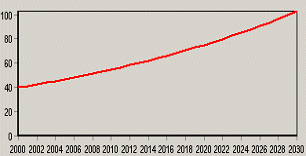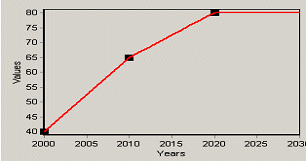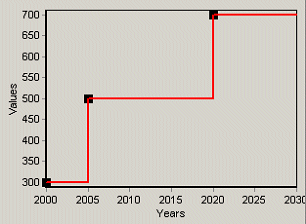# Examples of Expressions

 Type of Expression. Description Example Syntax and Graph Simple Number Calculates a constant value in all scenario years 3.1415 Simple Formula Calculates a constant value in all scenario years 0.1 * 5970 Growth Rate Calculates exponential growth over time from a Current Accounts value. NB: only valid in scenario expressions - not in Current Account expressions. Growth(3.2%)Interpolation Calculates straight-line change between specified pairs of data years and values. An optional final parameter lets you specify an exponential growth rate after the last data year. The function can include any number of year/value pairs, which need to be entered in ascending chronological order. Notice that the value parameters in this function can themselves be specified as mathematical functions. Interp(2000, 40, 2010, 65, 2020, 80)Interp(2000, 0.9 * BaseYearValue, 2030, 0.7 * BaseYearValue) Step Similar to the Interpolation function except that it calculates discrete changes between specified pairs of data years and values. Step(2000, 300, 2005, 500, 2020, 700)Remainder Calculates remaining value in one branch by subtracting values of all other neighboring branches from the function parameter. This function is useful for “share” branches, for example where you want to specify some branches as changing percentage share, and have one branch account for the remaining share. Remainder(100) Branch and Variable References Any WEAP variable can be calculated as a function of another variable (with some restrictions) Weaping River:Headflow * 0.25 GrowthAs Calculates a value in any given year based on its previous year's value and the rate of growth in another named branch, raised to the power of an elasticity. GrowthAs(Drivers\Income,1.1)